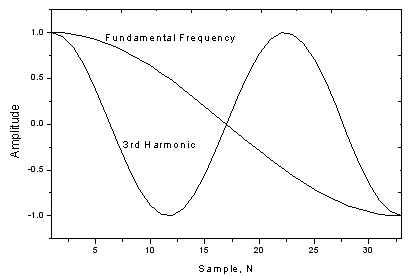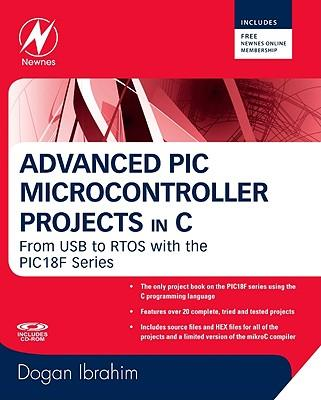# PIC Harmonic Distortion Meter

The 3rd harmonic distortion meter has been designed for measuring the quality of AC supply. The meter is built with a PIC18F2550 project board and the full wave rectifier front-end circuit. The AC power line, 220VAC is measured through the step down isolation transformer. The input signal to the 10-bit ADC is full wave rectified. The software performs DFT calculation finding the amplitude of the fundamental frequency and the 3rd harmonic. The distortion is computed by the ratio of the amplitude of the 3rd harmonic to the fundamental frequency. The meter has been tested with the square wave signal resulting 33% distortion. For low voltage AC utility, 220V, the reading showed approx. 3%. The meter can be applied for high voltage application with the appropriate signal conditioning.

Nowadays an increasing of the electronic devices having nonlinear characteristics are many used at home and office. Such devices mostly are computer based equipment with a low power factor switch mode power supply. The input circuit of the power supply uses a diode-capacitor at the front-end circuit. The current drawn is charging capacitor only near the peak voltage. Thus for a given feeder having finite impedance, there will be a lost from voltage dropped near the peak voltage resulting flattened top distortion of the AC voltage. To measure how high the distortion of AC voltage is, we may decompose it into the summation of sinusoid waves using DFT. The PIC harmonic distortion meter shows a method for finding the amplitude of the fundamental frequency and the 3rd harmonic. The reading shows percentage of the 3rd harmonic distortion.

READ  How to Wire a PIC Microcontroller?

To measure the waveshape distortion, we use the quantity of the Total Harmonic Distortion, THD (equation 1). THD is the ratio of the power of harmonic components to the power of fundamental frequency. Our concern is the voltage distortion, we can just find the sum of the rms of the harmonic components, Vn and the rms of the fundamental frequency, V1.Most of the harmonic problem is caused by the 3rd component. Since the 3rd harmonic is the 2nd highest energy from the fundamental component. So we interest to find only the 3rd harmonic distortion using equation 2.

We may decompose the periodic waveform, f(t) into the summation of a number of sinusoids waveform easily using the Discrete Fourier Transform (equation 3). A0 is the amplitude of DC components. For AC voltage waveform, A0 is zero.

For more detail: PIC Harmonic Distortion Meter

#### Current Project / Post can also be found using:

• harmonics recorder using pic